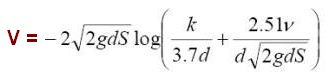# Colebrook White Equation Calculator

This calculator calculates the pipeline flow rate by using colebrook white equation method.

### Formula UsedColebrook White Equation

Where :

1. V – Mean flow velocity,
2. S – hydraulic gradient,
3. v – kinematic viscosity of water,
4. d – Internal diameter,
5. k – Roughness coefficient,
6. g = 9.81 m/s2,

Flow rate is calculated by multiplying mean velocity by area of section.

Note : While using below calculator, enter values as per units mentioned against individual input fields.

 Hydraulic Gradient (S) Kinematic Viscosity of Water (V) Internal Diameter (d) Area of Section (A) Colebrook Roughness Coefficient (k)